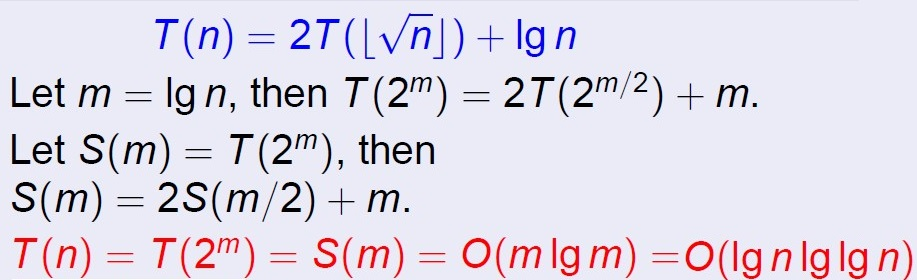程序算法艺术与实践:基础知识之函数的渐近的界

渐近符号

Θ 记号(读音:theta)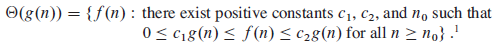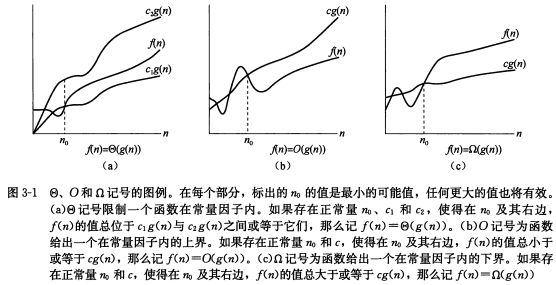O 符号

Θ 记号渐近地给出了一个函数的上界和下界。当只有 渐近 上界时，使用 O 记号。对一个函数 g ( n )，用 O ( g ( n ))表示一个函数集合：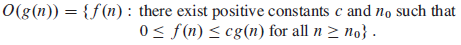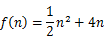， 则可以表示为 f(n) = O(n2)。证明：要使得 0 <= f(n) <= cg(n)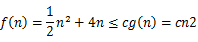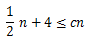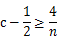o 记号

O 记号提供的渐近上界可能是也可能不是渐近紧确的。这里用 o 记号表示非渐近紧确的上界。 o ( g ( n ))的形式定义为集合：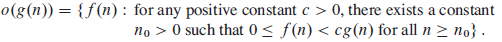O 记号与 o 记号的主要区别在于对 f ( n ) = O ( g ( n ))，界0 <= f ( n ) <= c g ( n )对某个常数 c > 0成立；但对 f ( n ) = o ( g ( n ))，界0 <= f ( n ) <= c g ( n )对所有常数 c > 0都成立。即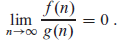Ω 记号

Ω 记号给出了函数的渐近下界。给定一个函数 g ( n )，用 Ω ( g ( n ))表示一个函数集合：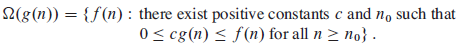ω 记号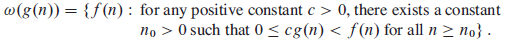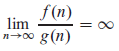函数间的比较

f ( n )和 g ( n )是渐近正值函数。

• f ( n ) = Θ ( g ( n ))和 g ( n ) = Θ ( h ( n )) 蕴含 f ( n ) = Θ ( h ( n ))
• f ( n ) = O ( g ( n ))和 g ( n ) = O ( h ( n )) 蕴含 f ( n ) = O ( h ( n ))
• f ( n ) = Ω ( g ( n ))和 g ( n ) = Ω ( h ( n )) 蕴含 f ( n ) = Ω ( h ( n ))
• f ( n ) = o ( g ( n ))和 g ( n ) = o ( h ( n )) 蕴含 f ( n ) = o ( h ( n ))
• f ( n ) = ω ( g ( n ))和 g ( n ) = ω ( h ( n )) 蕴含 f ( n ) = ω ( h ( n ))

• f ( n ) = Θ ( f ( n ))
• f ( n ) = O ( f ( n ))
• f ( n ) = Ω ( f ( n ))

• f ( n ) = Θ ( g ( n ))当且仅当 g ( n ) = Θ ( f ( n ))

• f ( n ) = O ( g ( n ))当且仅当 g ( n ) = Ω ( f ( n ))
• f ( n ) = o ( g ( n ))当且仅当 g ( n ) = ω ( f ( n ))

求解递归式的方法。

递归树法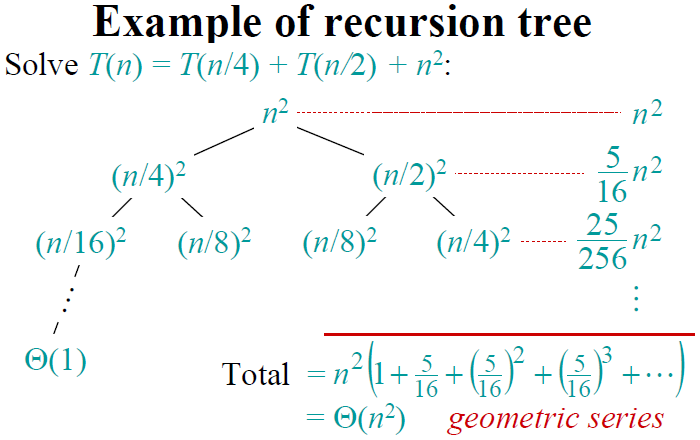主定理方法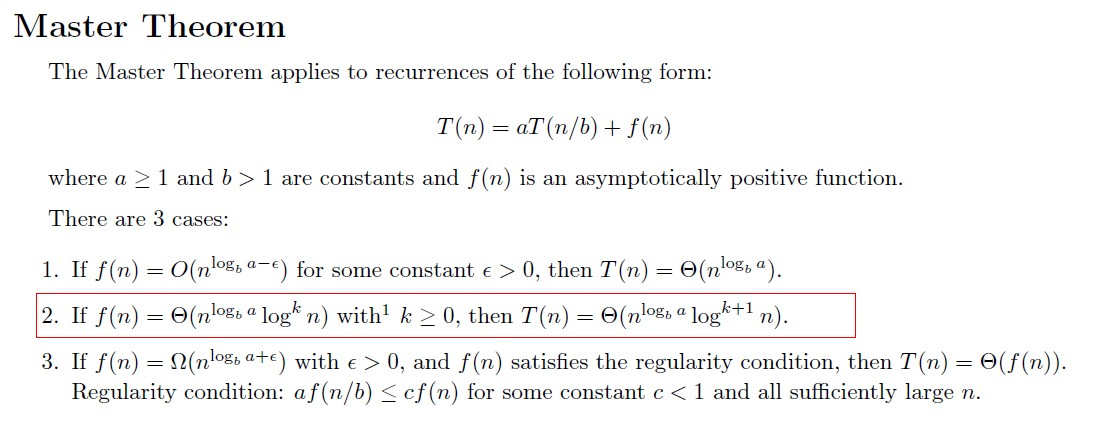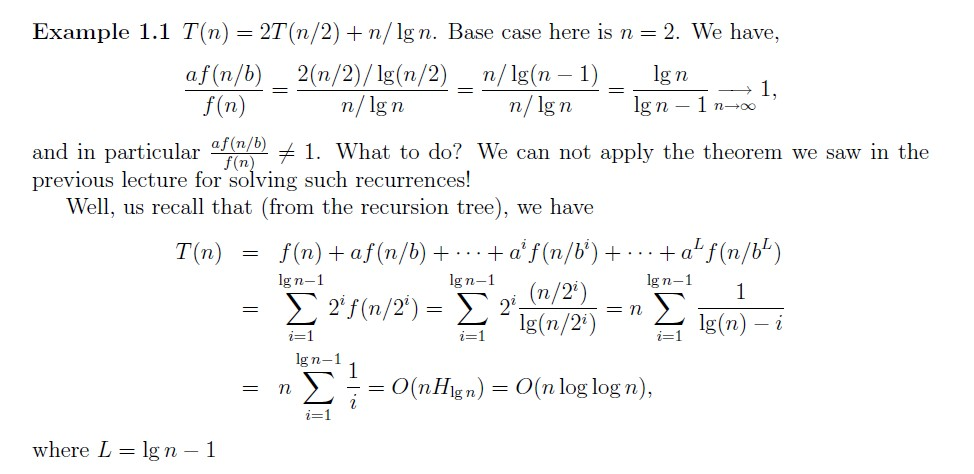T（n）=aT（n/b）+nk

T（n/b）=aT（n/b2）+（n/b）k

...